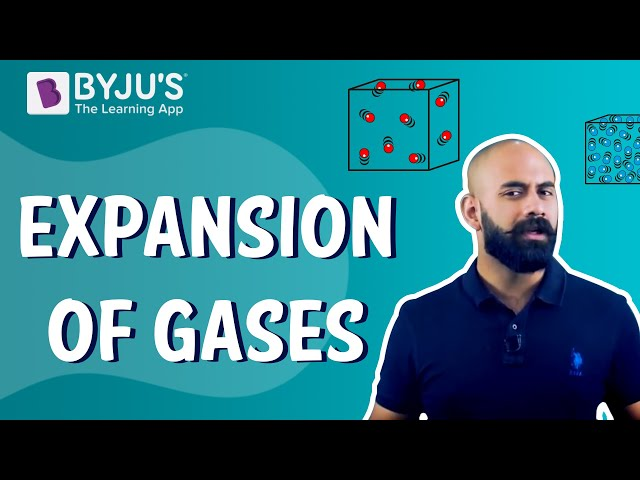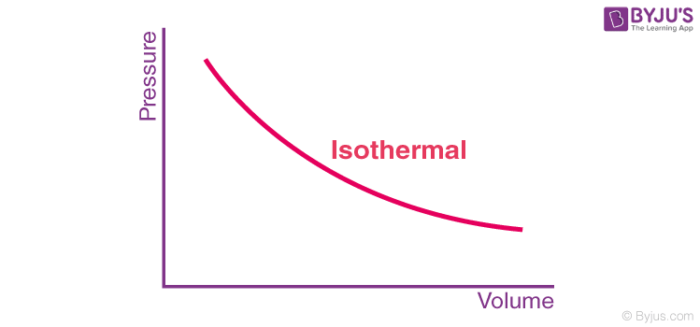Checkout JEE MAINS 2022 Question Paper Analysis : Checkout JEE MAINS 2022 Question Paper Analysis :

# Isothermal Expansion of an Ideal Gas

## Isothermal expansion

In an ideal gas, all the collisions between molecules or atoms are perfectly elastic and no intermolecular force of attraction exists in an ideal gas because of the molecules of an ideal gas move so fast, and they are so far away from each other that they do not interact at all. In the case of real gas, they have negligible intermolecular attractive forces. Ideal gas does not exist naturally. However, gases behave most ideally at high temperature and low-pressure conditions. The entire internal energy in an ideal gas is in the form of kinetic energy of the particles and any change in the internal energy results in a change of temperature.

## Recommended Videos

#### Expansion of Gases#### Reversible Isothermal Compression#### Isothermal Irreversible ExpansionNow that we have a basic understanding of the concept of an ideal gas let us see what the meaning of an isothermal expansion is and what is isothermal process. An isothermal process is a change in the system such that the temperature remains constant. In other words, in isothermal process ∆T = 0.Free expansion of a gas occurs when it is subjected to expansion in a vacuum (pex=0). During free expansion of an ideal gas, the work done is 0 be it a reversible or irreversible process.

It is known that the change in internal energy of a system is given as:

∆U = q + w—(1)

Where ∆U represents the change in internal energy, q is the heat given by the system and w is the work done on the system.

Depending upon the type of process the above equation can be written in different ways.

The work done in vacuum, w = pex∆V. Therefore, equation 1 can be given as:

∆U = q + pex∆V

If this process is done at constant volume then ∆V = 0. Thus,

∆U = Q

qv implies that the heat is supplied at a constant volume.

When an ideal gas is subjected to isothermal expansion (∆T = 0) in vacuum the work done w = 0 as pex=0. As determined by Joule experimentally q =0, thus ∆U = 0.

For isothermal reversible and irreversible changes; equation 1 can be expressed as:

• Isothermal reversible change: q = -w = pex(Vf-Vi)
• Isothermal reversible change: q = -w = nRTln (Vf/Vi) = 2.303 nRT log (Vf/Vi)